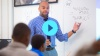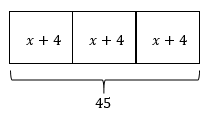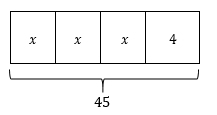# Equations and Inequalities

Students solve equations and inequalities with rational numbers, and encounter real-world situations that can be modeled and solved using equations and inequalities.

Math

Unit 4

## Unit Summary

In Unit 4, 7th grade students continue to build on the last two units by solving equations and inequalities with rational numbers. They use familiar tape diagrams as a way to visually model situations in the form $$px+q=r$$ and $$p(x+q)=r$$. These tape diagrams offer a pathway to solving equations using arithmetic, which students compare to a different approach of solving equations algebraically. Throughout the unit, students encounter word problems and real-world situations, covering the full range of rational numbers, that can be modeled and solved using equations and inequalities (MP.4). As they work with equations and inequalities, they build on their abilities to abstract information with symbols and to interpret those symbols in context (MP.2). Students also practice solving equations throughout the unit, ensuring they are working towards fluency which is an expectation in 7th grade.

In 6th grade, students understood solving equations and inequalities as a process of finding the values that made the equation or inequality true. They wrote and solved equations in the forms $$x+p=q$$ and $$px=q$$, using nonnegative rational numbers. In seventh grade, students reach back to recall these concepts and skills in order to solve one- and two-step equations and inequalities with rational numbers including negatives.

In 8th grade, students explore complex multi-step equations; however, they will discover that these multi-step equations can be simplified into forms that are familiar to what they’ve seen in 7th grade. In 8th grade, students will also investigate situations that result in solutions such as 5 = 5 or 5 = 8, and they will extend their understanding of solution to include no solution and infinite solutions.

Pacing: 16 instructional days (12 lessons, 3 flex days, 1 assessment day)

Fishtank Plus for Math

Unlock features to optimize your prep time, plan engaging lessons, and monitor student progress.## Assessment

The following assessments accompany Unit 4.

### Pre-Unit

Have students complete the Pre-Unit Assessment and Pre-Unit Student Self-Assessment before starting the unit. Use the Pre-Unit Assessment Analysis Guide to identify gaps in foundational understanding and map out a plan for learning acceleration throughout the unit.

### Mid-Unit

Have students complete the Mid-Unit Assessment after lesson 4.

### Post-Unit

Use the resources below to assess student understanding of the unit content and action plan for future units.

Expanded Assessment Package

Use student data to drive your planning with an expanded suite of unit assessments to help gauge students’ facility with foundational skills and concepts, as well as their progress with unit content.

## Unit Prep

### Intellectual Prep

Unit Launch

Prepare to teach this unit by immersing yourself in the standards, big ideas, and connections to prior and future content. Unit Launches include a series of short videos, targeted readings, and opportunities for action planning.#### Internalization of Standards via the Post-Unit Assessment

• Take the Post-Unit Assessment. Annotate for:
• Standards that each question aligns to
• Strategies and representations used in daily lessons
• Relationship to Essential Understandings of unit
• Lesson(s) that Assessment points to

#### Internalization of Trajectory of Unit

• Read and annotate the Unit Summary.
• Notice the progression of concepts through the unit using the Lesson Map.
• Essential Understandings
• Connection to Post-Unit Assessment questions
• Identify key opportunities to engage students in academic discourse. Read through our Teacher Tool on Academic Discourse and refer back to it throughout the unit.

#### Unit-Specific Intellectual Prep

 Model Example Tape diagram and equations $$3(x+4)=45$$$$3x+4=45$$### Essential Understandings

• Equations and inequalities are powerful tools that can be used to model and solve real-world situations with unknown quantities.
• Equations can be solved by reasoning about the arithmetic needed to uncover the value of the unknown. Equations can also be solved algebraically by using properties of operations and equality.
• Inequalities have infinite solutions, which can be represented graphically on a number line. In context, these solutions are sometimes constrained by what makes sense for the situation; for example, if solving for the maximum number of people who can fit onto a boat, the solution set would be limited to positive integers.

### Vocabulary

equation

inequality

solution

substitution

tape diagram

To see all the vocabulary for Unit 4, view our 7th Grade Vocabulary Glossary.

## Lesson Map

Topic A: Solving and Modeling with Equations

Topic B: Solving and Modeling with Inequalities

## Common Core Standards

Key

Major Cluster

Supporting Cluster

### Core Standards

#### Expressions and Equations

• 7.EE.B.3 — Solve multi-step real-life and mathematical problems posed with positive and negative rational numbers in any form (whole numbers, fractions, and decimals), using tools strategically. Apply properties of operations to calculate with numbers in any form; convert between forms as appropriate; and assess the reasonableness of answers using mental computation and estimation strategies. For example: If a woman making $25 an hour gets a 10% raise, she will make an additional 1/10 of her salary an hour, or$2.50, for a new salary of $27.50. If you want to place a towel bar 9 3/4 inches long in the center of a door that is 27 1/2 inches wide, you will need to place the bar about 9 inches from each edge; this estimate can be used as a check on the exact computation. • 7.EE.B.4 — Use variables to represent quantities in a real-world or mathematical problem, and construct simple equations and inequalities to solve problems by reasoning about the quantities. • 7.EE.B.4.A — Solve word problems leading to equations of the form px + q = r and p(x + q) = r, where p, q, and r are specific rational numbers. Solve equations of these forms fluently. Compare an algebraic solution to an arithmetic solution, identifying the sequence of the operations used in each approach. For example, the perimeter of a rectangle is 54 cm. Its length is 6 cm. What is its width? • 7.EE.B.4.B — Solve word problems leading to inequalities of the form px + q > r or px + q < r, where p, q, and r are specific rational numbers. Graph the solution set of the inequality and interpret it in the context of the problem. For example: As a salesperson, you are paid$50 per week plus $3 per sale. This week you want your pay to be at least$100. Write an inequality for the number of sales you need to make, and describe the solutions.

• 6.EE.B.5
• 6.EE.B.7
• 6.EE.B.8

• 8.EE.C.7
• 8.EE.C.8

### Standards for Mathematical Practice

• CCSS.MATH.PRACTICE.MP1 — Make sense of problems and persevere in solving them.

• CCSS.MATH.PRACTICE.MP2 — Reason abstractly and quantitatively.

• CCSS.MATH.PRACTICE.MP3 — Construct viable arguments and critique the reasoning of others.

• CCSS.MATH.PRACTICE.MP4 — Model with mathematics.

• CCSS.MATH.PRACTICE.MP5 — Use appropriate tools strategically.

• CCSS.MATH.PRACTICE.MP6 — Attend to precision.

• CCSS.MATH.PRACTICE.MP7 — Look for and make use of structure.

• CCSS.MATH.PRACTICE.MP8 — Look for and express regularity in repeated reasoning.

Unit 3

Numerical and Algebraic Expressions

Unit 5

Percent and Scaling

## Request a Demo

See all of the features of Fishtank in action and begin the conversation about adoption.

Yes

No

### Any other information you would like to provide about your school?